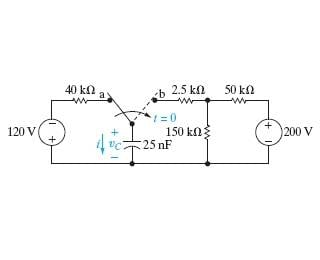# Current Through a Capacitor in a RC Circuit

• Engineering
• Drakkith
In summary, the current through the capacitor is estimated to be 85 mA, the current through the resistor is estimated to be -120 V, and the voltage across the capacitor is estimated to be -103.125 V.f

Mentor
2022 Award

## Homework Statement

Find ##i(0^+) ## and ##i(t), t≥0^+#### The Attempt at a Solution

[/B]
I'm having trouble finding the current through this capacitor. I tried using a KVL loop for ##t=0^+##, but I'm doing it incorrectly or something.

Earlier in the problem I found: ##v_c(0^+) = -120 V##
Sign convention for resistors: Positive on the top for the 150k resistor, positive on the right side for the other 2.

KVL1: Starting at node ##b## and moving clockwise with current ##i_1## :
##-2.5ki_1+150k(i_1-i_2)-v_c=0##
##-2.5ki_1+150ki_1-150ki_2-(-120)=0##
##147.5ki_1-150ki_2=-120##

KVL2: Starting just to the left of the 50k resistor and moving clockwise:
##-50ki_2+200-150k(i_2-i_1)=0##
##-50ki_2-150ki_2+150ki_1=-200##
##150ki_1-200ki_2=-200##

Solving, I get:
##i_1=0.85 mA##
##i_2=1.6mA##

##i=-i_1=-0.85 mA##

However, this is appears to be incorrect.

#### Attachments

Earlier in the problem I found: vc(0+)=−120V
Looks correct.
I tried using a KVL loop
For me, the KVL equations are generally less intuitive and move complicated. Not to make extra work for you, but can you write the KCL equation for the main node in the circuit for t>0 and see if that makes it easier to solve?

For me, the KVL equations are generally less intuitive and move complicated. Not to make extra work for you, but can you write the KCL equation for the main node in the circuit for t>0 and see if that makes it easier to solve?

Sure. From the main node, ##i## goes left, ##i_1## goes down, and ##i_2## goes right.
##i+i_1+i_2=0##
##\frac{v-v_c}{2.5}+\frac{v}{150k}+\frac{v-200}{50k}=0##
Multiplying both sides by 150k:
##60v-60v_c+v+3v-600=0##
##64v-60(-120)=600##
##64v+7200=600##
##64v=-6600##
##v=-103.125 V##

##i=\frac{v-v_c}{2500}=6.75 mA##
Which is correct...

##i(t)## is then: ##i(t)=6.75e^{(1000t)} mA##

Why in the world did one method work but not the other?

Which is correct...
Yahoo!Why in the world did one method work but not the other?
Both methods should work, but at least for me, the KVL equations are much less intuitive and therefore much easier to make a mistake when using them. I'll leave it to other (smarter) members (EDIT -- like vela) to find the small typo in your work with the KVL equations...Last edited:
•cnh1995 and Drakkith
Looks like the signs aren't consistent in your KVL equations. If you're going clockwise around the left loop, for example, you should get
$$-2500i_1 + 150000(i_2-i_1) + v_c = 0.$$ In the second KVL equation, the sign on 200 should be negative since you're moving from higher potential to lower potential as you go clockwise around the loop.

•berkeman
Looks like the signs aren't consistent in your KVL equations. If you're going clockwise around the left loop, for example, you should get
$$-2500i_1 + 150000(i_2-i_1) + v_c = 0.$$ In the second KVL equation, the sign on 200 should be negative since you're moving from higher potential to lower potential as you go clockwise around the loop.

Isn't that already consistent though? Moving from positive to negative voltage across a resistor (moving from higher to lower potential), the sign is +, whereas it's - when moving from negative to positive (as in the ##-2500i_1##).

Which way are you assuming the currents ##i_1## and ##i_2## flow? If it's clockwise around the loops, then according to your sign convention, the first term should be ##+2500i_1##.